# Statistical Functions

This chapter gives an overview of some very useful statistical functions in Excel.

### Average

To calculate the average of a group of numbers, use the AVERAGE function.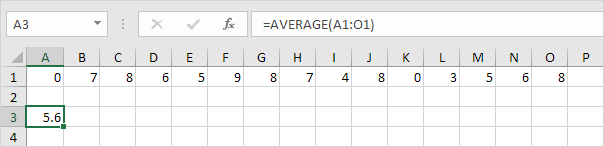### Averageif

To average cells based on one criteria, use the AVERAGEIF function. For example, to calculate the average excluding zeros.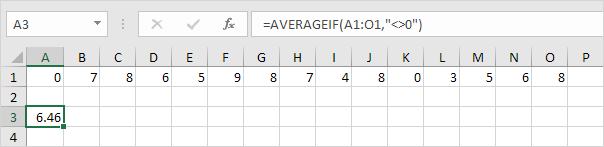### Median

To find the median (or middle number), use the MEDIAN function.Check:### Mode

To find the most frequently occurring number, use the MODE function.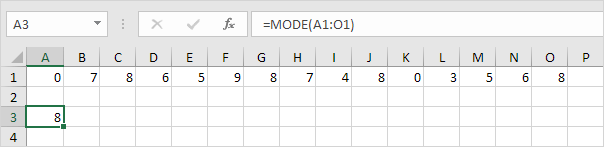### Standard Deviation

To calculate the standard deviation, use the STEDV function.### Min

To find the minimum value, use the MIN function.### Max

To find the maximum value, use the MAX function.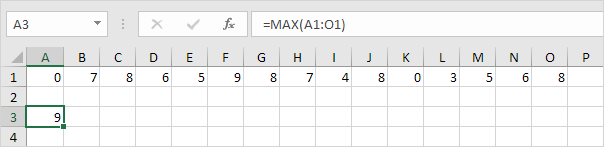### Large

To find the third largest number, use the following LARGE function.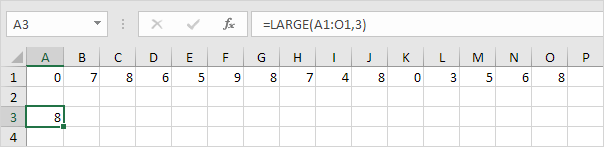Check: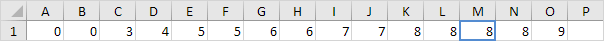### Small

To find the second smallest number, use the following SMALL function.Check:Tip: Excel can generate most of these results with the click of a button. Our Descriptive Statistics example shows you how.

Previous articleXML in Excel
Next articleArray Formulas in Excel